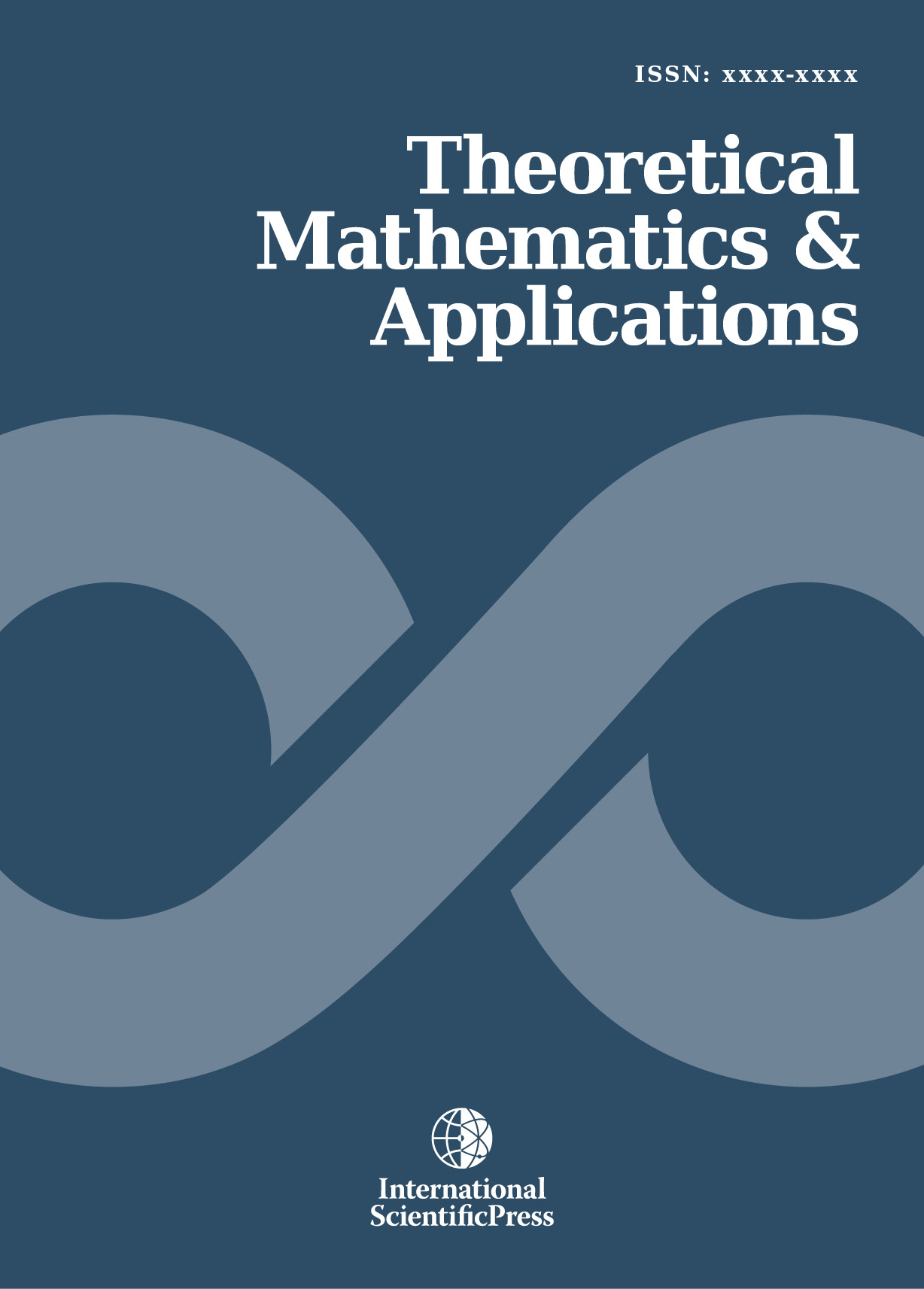# Theoretical Mathematics & Applications

#### Numerical solution of Fractional Integro-Differential Equations by Least Squares Method and Shifted Chebyshev Polynomials of the third kind method

•[ Download ]
• Times downloaded: 1086
• Abstract

In this paper, an implementation of an efficient numerical method of linear fractional integro-differential equations (LFIDEs) by least squares method with aid of shifted Chebyshev polynomials of the third kind method. The fractional derivative is described in the Caputo sense. The method is based upon shifted Chebyshev polynomials of the third kind approximations is introduced. Some numerical examples are presented to illustrate the theoretical results and compared with the results obtained by other numerical methods. We have computed the numerical results using Mathematica 9 programming.ISSN: 1792-9687 (Print)
1792-9709 (Online)